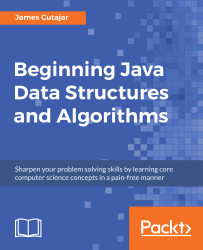•#### Beginning Java Data Structures and Algorithms#### Overview of this book

Learning about data structures and algorithms gives you a better insight on how to solve common programming problems. Most of the problems faced everyday by programmers have been solved, tried, and tested. By knowing how these solutions work, you can ensure that you choose the right tool when you face these problems. This book teaches you tools that you can use to build efficient applications. It starts with an introduction to algorithms and big O notation, later explains bubble, merge, quicksort, and other popular programming patterns. You’ll also learn about data structures such as binary trees, hash tables, and graphs. The book progresses to advanced concepts, such as algorithm design paradigms and graph theory. By the end of the book, you will know how to correctly implement common algorithms and data structures within your applications.
Title PagePackt UpsellContributorsPrefaceFree Chapter
Algorithms and ComplexitiesSorting Algorithms and Fundamental Data StructuresHash Tables and Binary Search TreesString Matching AlgorithmsGraphs, Prime Numbers, and Complexity ClassesOther Books You May EnjoyIndex## Getting Started with Divide and Conquer Algorithms

In Chapter 2, Sorting Algorithms and Fundamental Data Structures, we introduced, among other sorting algorithms, merge and quick sort. A peculiarity of both algorithms is that they divide the problem into subproblems that are smaller instances of the same, solve each one of the subproblems recursively, and then combine the solutions to the subproblems into the solution for the original problem. This strategy forms the basis of the divide and conquer paradigm.

### The Divide and Conquer Approach

In a divide and conquer approach, we solve a problem recursively, applying the following three steps at each level of recursion:

• Divide the problem into more than one subproblems that are smaller instances of the same problem.
• Conquer the subproblems by solving them recursively. Eventually, the subproblem sizes are small enough for them to be solved in a straightforward manner.
• Combine the solutions to the subproblems in the solution for the original problem...